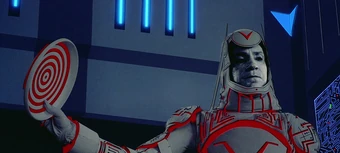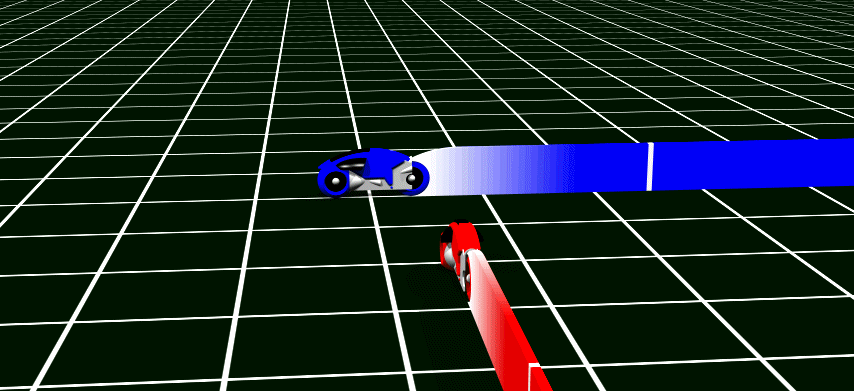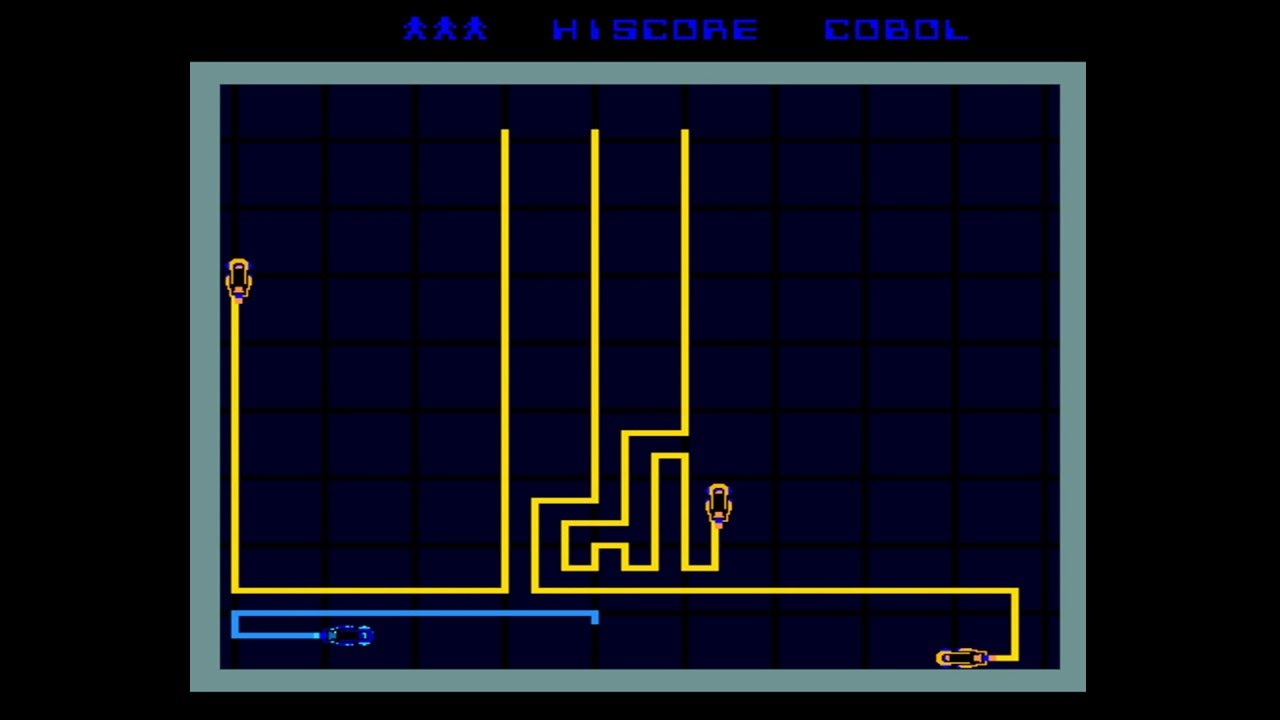So, a TRON Light Cycle style of game... Remember TRON?I need my Light Cycles to detect the "light walls" that the Light Cycles generate as they speed around the Game Grid in order for them to queue_free() correctly... :DSo far, the closest I've gotten to the effect I'm seeking is by generating a Line2D via an array of points based on the cycle's positions throughout the _process() function. The cycle should crash into the Line2d/"light wall" if any of those positions/Line2D points are found in the array twice.The problem I'm running into now, is that the positions/Line2D points of Player 1's Light Cycle are almost always a fraction off from Player 2's Light Cycle. So, Player 1 can crash into its own wall, but not into Player 2's wall, and vice versa.. or at least, it rarely occurs.

Since the Vectors are floats, I tried converting the array's points to int, or rounding the floats up or down, etc... This allows the cycles to detect their opponents walls more frequently, but still, not every time. However, this leads to other problems (the Light Cycle will frequently round to the same position within one or two steps of its move _and _slide() sequence.. or sometimes it will skip over its own previous positions.I would think this should be easy to do, but I'm such a noob, it's taking me forever to get this mechanic implemented correctly. So, is there some reasonable way I can do this? Am I going about it completely wrong? Is there a collision or signal I can use? I tried the CollisionPolygon2D node, but that drew a whole shape, not just the line...

Sorry if this is unclear. Please let me know if I can clarify or if I should post some code or pics of what i've got so far..

in Engine

I think this method will work.
subdivide your level into cells and for each individual cell can have an int value.
0 means nothing , 1 means player 1, 2 means player 2.

``````var cell_count = vector2(400,400)   #increase it for higher precision
var world_size = Vector2(1280,720)  #set size of your map in pixels

onready var ratio = world_size / cell_count

var matrix = []

#create 2d array and set it to 0
for x in range(cell_count.x):
matrix.append([])
for y in range(cell_count.y):
matrix[x].append(0)

func _process(_delta):
var cpos = player1.position / ratio

if matrix[int(cpos.x)][int(cpos.y)] == 1:
#suicide?
elif matrix[int(cpos.x)][int(cpos.y)] == 2:
#killed by player 2
else:
matrix[int(cpos.x)][int(cpos.y)] = 1

#player 2
cpos = player2.position / ratio

if matrix[int(cpos.x)][int(cpos.y)] == 1:
#killed by player 1
elif matrix[int(cpos.x)][int(cpos.y)] == 2:
#suicide?
else:
matrix[int(cpos.x)][int(cpos.y)] = 2
``````

I haven't tested but this should work

by (731 points)

Thanks, It should work. If it doesn't, at least you've given me some ideas. Good idea!